## Decimals

Fractions also may be written in decimal form (decimal fractions) as either terminating, coming to an end (for example 0.3 or 0.125), or having an infinite (never ending) repeating pattern (for example (0.666… or 0.1272727…).

#### Changing terminating decimals to fractions

To change terminating decimals to fractions, remember that all numbers to the right of the decimal point are fractions with denominators of only 10, 100, 1000, 10,000, and so forth. Next, use the technique of read it, write it, and reduce it.

##### Example 1

Change the following to fractions in lowest terms.

1.2.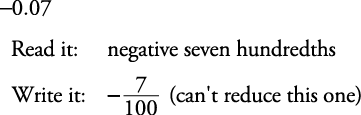All rules for signed numbers also apply to operations with decimals.

#### Changing fractions to decimals

To change a fraction to a decimal, simply do what the operation says. In other wordsmeans 13 divided by 20. So do just that (insert decimal points and zeros accordingly).

##### Example 2

Change to decimals.

1.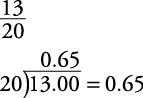2.#### Changing infinite repeating decimals to fractions

Infinite repeating decimals usually are represented by putting a line over (sometimes under) the shortest block of repeating decimals. This line is called a vinculum. So you would write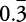to indicate .333 …to indicate .515151 …to indicate –2.1474747 …

Notice that only the digits under the vinculum are repeated.

Every infinite repeating decimal can be expressed as a fraction.

##### Example 3

Find the fraction represented by the repeating decimal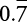.Because 10 n and n have the same fractional part, their difference is an integer.Therefore,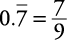##### Example 4

Find the fraction represented by the repeating decimal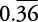.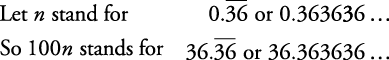Because 100 n and 1 n have the same fractional part, their difference is an integer.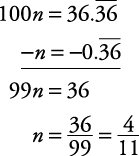Therefore,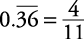##### Example 5

Find the fraction represented by the repeating decimal.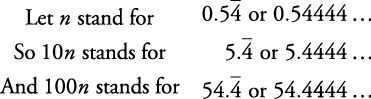Because 100 n and 10 n have the same fractional part, their difference is an integer.Therefore,